# Matrix Calculator

Set the size of the matrix and input the values in the matrix calculator. Choose the operation to be performed and click calculate.

Give Us Feedback

This online matrix solver is used to perform three main mathematical operations (i.e +, -, x) on the matrices up to the size of 5x5. It gives a detailed and comprehensive step-by-step solution as well.

## What is a matrix?

A matrix, in mathematics, represents the property or quantity of an object. Its structure is rectangular and contains a table of symbols or expressions.

An example of a matrix is: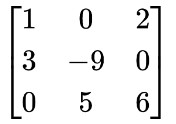## How to solve matrices?

The sum and difference of the two matrices are simple. The first entry of the first matrix is added or subtracted from the first entry of the second matrix.

Add matrices A, B, and C.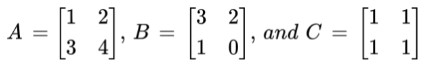Solution:

Add A and B first. Then add matrix C in the resultant matrix.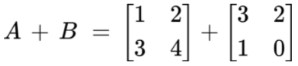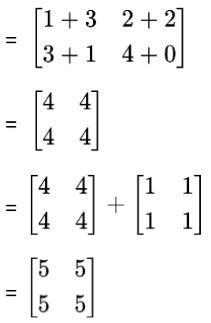Example - Matrix subtraction

Let's subtract matrix B from matrix A.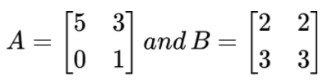Solution:

Subtract each corresponding element of both matrices.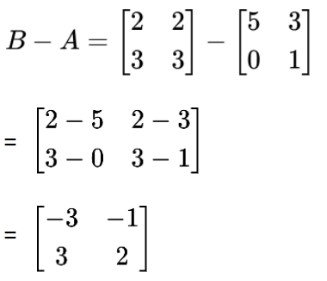Example - Matrix multiplication

The process of multiplying two matrices requires different techniques. The first row of matrix A is multiplied by the first column of matrix B.

Multiply matrix A by matrix B, where;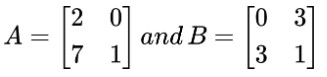Solution: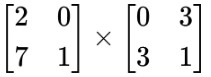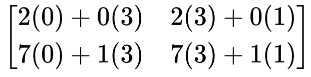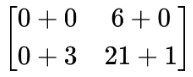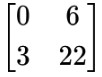Use the matrices calculator above to solve matrices.

### Math Tools